# forecast

Forecast identified model output

## Syntax

``yf = forecast(sys,PastData,K)``
``yf = forecast(sys,PastData,K,FutureInputs) ``
``yf = forecast(___,opts)``
``````[yf,x0,sysf] = forecast(___)``````
``````[yf,x0,sysf,yf_sd,x,x_sd] = forecast(___)``````
``forecast(sys,PastData,K,___)``
``forecast(sys,Linespec,PastData,K,___)``
``forecast(sys1,...,sysN,PastData,K,___)``
``forecast(sys1,Linespec1,...,sysN,LinespecN,PastData,K,___)``

## Description

example

````yf = forecast(sys,PastData,K)` forecasts the output of an identified time series model `sys`, `K` steps into the future using past measured time-domain data, `PastData`. `PastData` can be a timetable, a comma-separated input/output matrix pair, or a time-domain `iddata` object.`forecast` performs prediction into the future, in a time range beyond the last instant of measured data. In contrast, the `predict` command predicts the response of an identified model over the time span of measured data. Use `predict` to determine if the predicted result matches the observed response of an estimated model. If `sys` is a good prediction model, consider using it with `forecast`.```

example

````yf = forecast(sys,PastData,K,FutureInputs) ` uses the future values of the inputs, `FutureInputs`, to forecast the response of an identified model with input channels.```

example

````yf = forecast(___,opts)` uses the option set, `opts`, to specify additional forecast options. Use `opts` with any of the previous input argument combinations.```

example

``````[yf,x0,sysf] = forecast(___)``` also returns the estimated values for initial states, `x0`, and a forecasting model, `sysf`, and can include any of the previous input argument combinations.```

example

``````[yf,x0,sysf,yf_sd,x,x_sd] = forecast(___)``` also returns estimated standard deviation of the output, `yf_sd`, state trajectory, `x`, and standard deviation of the trajectory, `x_sd`. Use with any of the previous input argument combinations.```

example

````forecast(sys,PastData,K,___)` plots the forecasted output. Use with any of the previous input argument combinations.To change display options, right-click the plot to access the context menu. For example, to view the estimated standard deviation of the forecasted output, select Confidence Region from the context menu. For more details about the menu, see Tips.```

example

````forecast(sys,Linespec,PastData,K,___)` uses `Linespec` to specify the line type, marker symbol, and color.```
````forecast(sys1,...,sysN,PastData,K,___)` plots the forecasted outputs for multiple identified models. `forecast` automatically chooses colors and line styles.```
````forecast(sys1,Linespec1,...,sysN,LinespecN,PastData,K,___)` uses the line type, marker symbol, and color specified for each system.```

## Examples

collapse all

Forecast the values of a sinusoidal signal using an AR model.

Generate and plot data.

```data = iddata(sin(0.1*[1:100])',[]); plot(data)```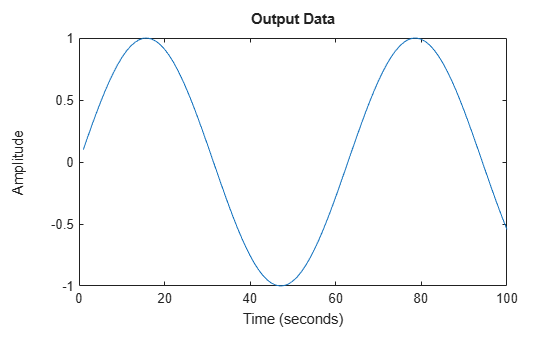Fit an AR model to the sine wave.

`sys = ar(data,2);`

Forecast the values into the future for a given time horizon.

```K = 100; p = forecast(sys,data,K);```

`K` specifies the forecasting time horizon as 100 samples. `p` is the forecasted model response.

Plot the forecasted data.

`plot(data,'b',p,'r'), legend('measured','forecasted')`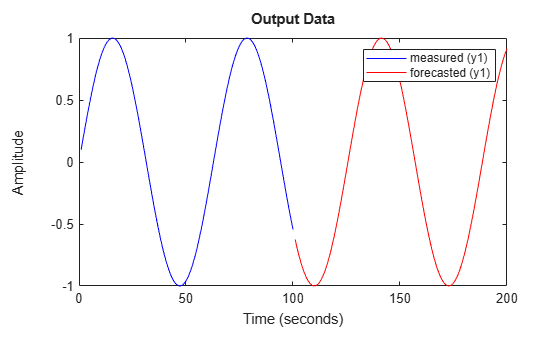Alternatively, plot the forecasted output using the syntax `forecast(sys,data,K)`.

Obtain past data, and identify a time series model.

```load sdata9 tt9 past_data = tt9.y(1:50); model = ar(tt9,4);```

`tt9` is an `iddata` object that contains measured output only.

`model` is an `idpoly` time series model.

Specify initial conditions for forecasting.

`opt = forecastOptions('InitialCondition','e');`

Plot the forecasted system response for a given time horizon.

```K = 100; forecast(model,past_data,K,opt); legend('Measured','Forecasted')```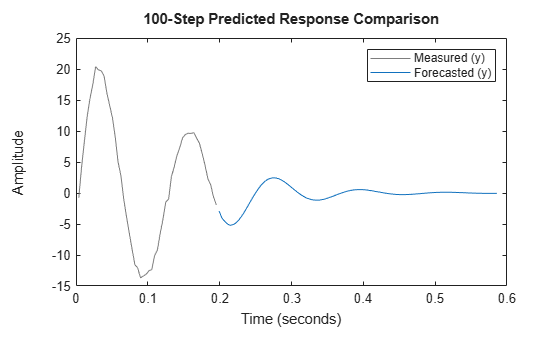Obtain past data, and identify a time series model.

```load iddata9 z9 past_data = z9.OutputData(1:50); model = ar(z9,4);```

`z9` is an `iddata` object that contains measured output only.

Plot the forecasted system response for a given time horizon as a red dashed line.

```K = 100; forecast(model,'r--',past_data,K);```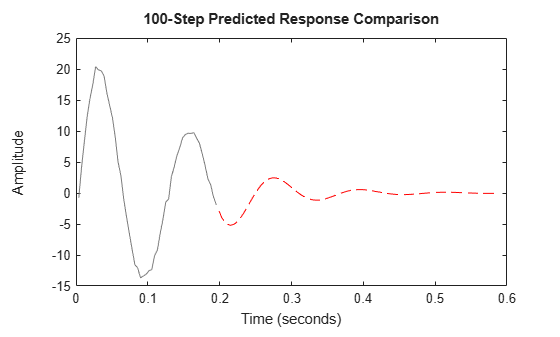The plot also displays the past data by default. To change display options, right-click the plot to access the context menu. For example, to view the estimated standard deviation of the forecasted output, select ConfidenceRegion from the context menu. To specify number of standard deviations to plot, double-click the plot and open the Property Editor dialog box. In the dialog box, in the Options tab, specify the number of standard deviations in Confidence Region for Identified Models. The default value is `1` standard deviation.

Obtain past data, future inputs, and an identified linear model.

```load iddata1 z1 z1 = iddata(cumsum(z1.y),cumsum(z1.u),z1.Ts,'InterSample','foh'); past_data = z1(1:100); future_inputs = z1.u(101:end); sys = polyest(z1,[2 2 2 0 0 1],'IntegrateNoise',true);```

`z1` is an `iddata` object that contains integrated data. `sys` is an `idpoly` model. `past_data` contains the first 100 data points of `z1`.

`future_inputs` contains the last 200 data points of `z1`.

Forecast the system response into the future for a given time horizon and future inputs.

```K = 200; [yf,x0,sysf,yf_sd,x,x_sd] = forecast(sys,past_data,K,future_inputs);```

`yf` is the forecasted model response, and `yf_sd` is the standard deviation of the output. `x0` is the estimated value for initial states, and `sysf` is the forecasting state-space model. Also returned are the state trajectory, `x`, and standard deviation of the trajectory, `x_sd`.

Plot the forecasted response.

```UpperBound = iddata(yf.OutputData+3*yf_sd,[],yf.Ts,'Tstart',yf.Tstart); LowerBound = iddata(yf.OutputData-3*yf_sd,[],yf.Ts,'Tstart',yf.Tstart); plot(past_data(:,:,[]),yf(:,:,[]),UpperBound,'k--',LowerBound,'k--') legend({'Measured','Forecasted','3 sd uncertainty'},'Location','best')```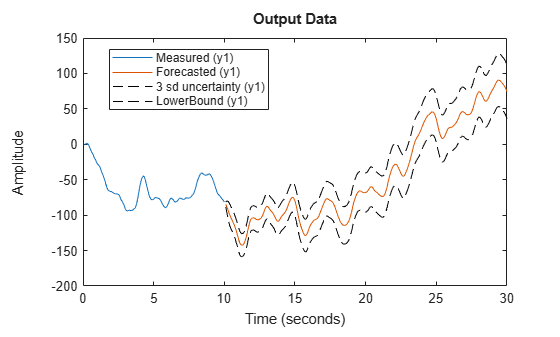Plot the state trajectory.

```t = z1.SamplingInstants(101:end); subplot(3,1,1) plot(t,x(:,1),t,x(:,1)+3*x_sd(:,1),'k--',t,x(:,1)-3*x_sd(:,1),'k--') title('X_1') subplot(3,1,2) plot(t, x(:,2),t,x(:,2)+3*x_sd(:,2),'k--',t, x(:,2)-3*x_sd(:,2),'k--') title('X_2') subplot(3,1,3) plot(t,x(:,3),t,x(:,3)+3*x_sd(:,3),'k--',t, x(:,3)-3*x_sd(:,3),'k--') title('X_3')```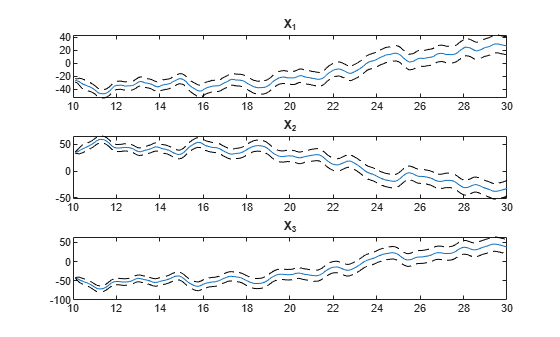The response uncertainty does not grow over the forecasting time span because of the specification of future inputs.

```load(fullfile(matlabroot,'toolbox','ident','iddemos','data','predprey2data')); z = iddata(y,[],0.1); set(z,'Tstart',0,'OutputUnit',{'Population (in thousands)',... 'Population (in thousands)'},'TimeUnit','Years');```

`z` is a two output time-series data set (no inputs) from a 1-predator 1-prey population. The population exhibits a decline in predator population due to crowding. The data set contains 201 data samples covering 20 years of evolution.

The changes in the predator (`y1`) and prey (`y2`) population can be represented as:

`${y}_{1}\left(t\right)={p}_{1}*{y}_{1}\left(t-1\right)+{p}_{2}*{y}_{1}\left(t-1\right)*{y}_{2}\left(t-1\right)$`

`${y}_{2}\left(t\right)={p}_{3}*{y}_{2}\left(t-1\right)-{p}_{4}*{y}_{1}\left(t-1\right)*{y}_{2}\left(t-1\right)-{p}_{5}*{y}_{2}\left(t-1{\right)}^{2}$`

The nonlinearity in the predator and prey populations can be fit using a nonlinear ARX model with custom regressors.

Use part of the data as past data.

`past_data = z(1:100);`

Specify the standard regressors.

```na = [1 0; 0 1]; nb = []; nk = [];```

Specify the custom regressors.

`C = {{'y1(t-1)*y2(t-1)'};{'y1(t-1)*y2(t-1)','y2(t-1)^2'}};`

Estimate a nonlinear ARX model using `past_data` as estimation data.

`sys = nlarx(past_data,[na nb nk],'idWaveletNetwork','CustomRegressors',C);`

Compare the simulated output of `sys` with measured data to ensure it is a good fit.

`compare(past_data,sys);`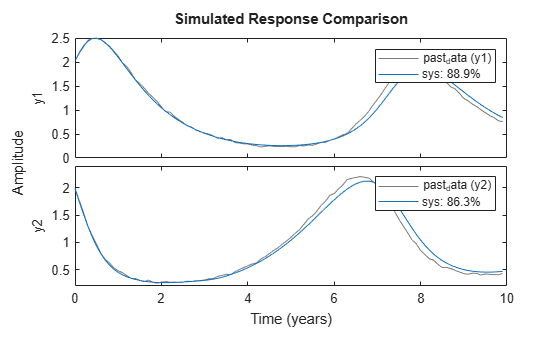Plot the forecasted output of `sys`.

```forecast(sys,past_data,101); legend('Measured','Forecasted');```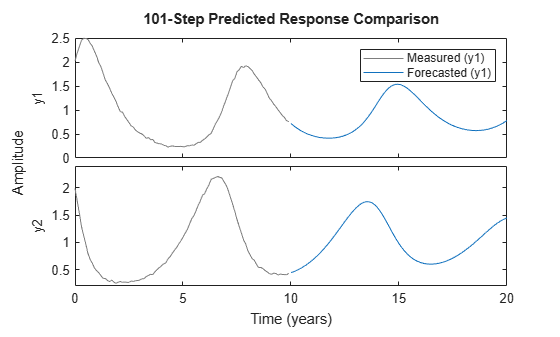Obtain past data, future inputs, and identified linear model.

```load iddata3 z3 past_data = z3(1:100); future_inputs = z3.u(101:end); sys = polyest(z3,[2 2 2 0 0 1]);```

Forecast the system response into the future for a given time horizon and future inputs.

```K = size(future_inputs,1); [yf,x0,sysf] = forecast(sys,past_data,K,future_inputs);```

`yf` is the forecasted model response, `x0` is the estimated value for initial states, and `sysf` is the forecasting state-space model.

Simulate the forecasting state-space model with inputs, `future_inputs`, and initial conditions, `x0`.

```opt = simOptions; opt.InitialCondition = x0; ys = sim(sysf,future_inputs(1:K),opt);```

Plot the forecasted and simulated outputs.

```t = yf.SamplingInstants; plot(t,yf.OutputData,'b',t,ys,'.r'); legend('Forecasted Output','Simulated Output')```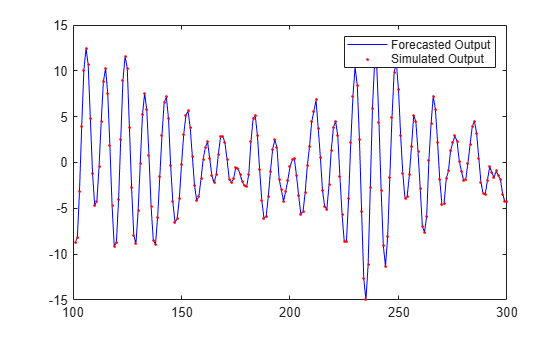Simulation of forecasting model, `sysf`, with inputs, `future_inputs`, and initial conditions, `x0`, yields the forecasted output, `yf`.

## Input Arguments

collapse all

Identified model whose output is to be forecasted, specified as one of the following:

If a model is unavailable, estimate `sys` from `PastData` using commands such as `ar`, `arx`, `armax`, `nlarx`, and `ssest`.

Measured uniformly sampled input/output data, specified as a `timetable`, a comma-separated pair of numeric input/output matrices, or an `iddata` object. The specification for `PastData` depends on the data type.

#### Timetable

Specify `PastData` as a `timetable` that uses a regularly spaced time vector. `tt` contains variables representing input and output channels.

#### Numeric Matrix

Specify `PastData` as one of the following:

• A comma-separated pair of real-valued matrices that contain the input and output time-domain signal values u,y. Specify the input matrix with the dimensions Ns-by-Nu and the output matrix with the dimensions Ns-by-Ny, where Nu is the number of inputs, Ny is the number of outputs, and Ns is the number of samples.

For time series data, specify `[]`,y.

Use matrix-based data only for discrete-time models.

#### Data Object

Specify data as an `iddata` object that contains the input and output data. For time-series data (no inputs), specify `PastData` as an `iddata` object with no inputs.

For more information about working with estimation data types, see Data Domains and Data Types in System Identification Toolbox.

Time horizon of forecasting, specified as a positive integer. The output, `yf`, is calculated `K` steps into the future, such that the prediction time horizon is `Ts*K`.

Future input values, specified as one of the following:

• `[]` — Future input values are assumed to be zero, or equal to input offset levels (if they are specified in `opts`). For time series models, specify as `[]`.

• `iddata` object — Specify as an `iddata` object with no outputs.

• K-by-Nu matrix of doubles — `K` is the forecast horizon, and Nu is the number of inputs.

If you have data from multiple experiments, you can specify a cell array of matrices, one for each experiment in `PastData`.

Forecast options, specified as a `forecastOptions` option set.

Line style, marker, and color, specified as a character vector. For example, `'b'` or `'b+:'`.

For more information about configuring `Linespec`, see the Linespec argument of `plot`.

## Output Arguments

collapse all

Forecasted response, returned as in the same form as `PastData`. `yf` is the forecasted response at times after the last sample time in `PastData`. `yf` contains data for the time interval `T0+(N+1:N+K)*T1`, where ```T0 = PastData.Tstart``` and `T1 = PastData.Ts`. N is the number of samples in `PastData`.

Estimated initial states at the start of forecasting, returned as a column vector of size equal to the number of states. Use `x0` with the forecasting model `sysf` to reproduce the result of forecasting by pure simulation.

If `PastData` is multi-experiment, `x0` is a cell array of size Ne, where Ne is the number of experiments.

When `sys` is not a state-space model (`idss`, `idgrey`, or `idnlgrey`), the definition of states depends on if `sys` is linear or nonlinear:

Forecasting model, returned as one of the following:

• Discrete-time `idss` — If `sys` is a discrete-time `idss` model, `sysf` is the same as `sys`. If `sys` is a linear model that is not a state-space model (`idpoly`, `idproc`, `idtf`), or is a continuous-time state-space model (`idss`, `idgrey`), `sys` is converted to a discrete-time `idss` model. The converted model is returned in `sysf`.

• `idnlarx`, `idnlhw`, or `idnlgrey`— If `sys` is a nonlinear model, `sysf` is the same as `sys`.

• Cell array of models — If `PastData` is multiexperiment, `sysf` is an array of Ne models, where Ne is the number of experiments.

Simulation of `sysf` using `sim`, with inputs, `FutureInputs`, and initial conditions, `x0`, yields `yf` as the output. For time-series models, `FutureInputs` is empty.

Estimated standard deviations of forecasted response, returned as a K-by-Ny matrix, where `K` is the forecast horizon, and Ny is the number of outputs. The software computes the standard deviation by taking into account the model parameter covariance, initial state covariance, and additive noise covariance. The additive noise covariance is stored in the `NoiseVariance` property of the model.

If `PastData` is multiexperiment, `yf_sd` is a cell array of size Ne, where Ne is the number of experiments.

`yf_sd` is empty if `sys` is a nonlinear ARX (`idnlarx`) or Hammerstein-Wiener model (`idnlhw`). `yf_sd` is also empty if `sys` does not contain parameter covariance information, that is if `getcov(sys)` is empty. For more information, see `getcov`.

Forecasted state trajectory, returned as a K-by-Nx matrix, where `K`, the forecast horizon and Nx is the number of states. `x` are the states of the forecasting model.

If `PastData` is multiexperiment, `x` is a cell array of size Ne, where Ne is the number of experiments.

If `sys` is linear model other than a state-space model (not `idss` or `idgrey`), then it is converted to a discrete-time state-space model, and the states of the converted model are calculated. If conversion of `sys` to `idss` is not possible, `x` is returned empty. For example, if `sys` is a MIMO continuous-time model with irreducible internal delays.

`x` is empty if `sys` is a nonlinear ARX (`idnlarx`) or Hammerstein-Wiener model (`idnlhw`).

Estimated standard deviations of forecasted states `x`, returned as a K-by-Ns matrix, where `K`, the forecast horizon and Ns is the number of states. The software computes the standard deviation by taking into account the model parameter covariance, initial state covariance, and additive noise covariance. The additive noise covariance is stored in the `NoiseVariance` property of the model.

If `PastData` is multiexperiment, `x_sd` is a cell array of size Ne, where Ne is the number of experiments.

If `sys` is linear model other than a state-space model (not `idss` or `idgrey`), then it is converted to a discrete-time state-space model, and the states and standard deviations of the converted model are calculated. If conversion of `sys` to `idss` is not possible, `x_sd` is returned empty. For example, if `sys` is a MIMO continuous-time model with irreducible internal delays.

`x_sd` is empty if `sys` is a nonlinear ARX (`idnlarx`) or Hammerstein-Wiener model (`idnlhw`).

## Tips

• Right-clicking the plot opens the context menu, where you can access the following options:

• Systems — Select systems to view forecasted output. By default, the forecasted output of all systems is plotted.

• Data Experiment — For multi-experiment data only. Toggle between data from different experiments.

• Characteristics — View the following data characteristics:

• Peak Value — View peak value of the data.

• Mean Value — View mean value of the data.

• Confidence Region — View the estimated standard deviation of the forecasted output. To specify number of standard deviations to plot, double-click the plot and open the Property Editor dialog box. Specify the number of standard deviations in the Options tab, in Confidence Region for Identified Models. The default value is `1` standard deviation.

The confidence region is not generated for nonlinear ARX and Hammerstein-Wiener models and models that do not contain parameter covariance information.

• Show Past Data — Plot the past output data used for forecasting. By default, the past output data is plotted.

• I/O Grouping — For datasets containing more than one input or output channel. Select grouping of input and output channels on the plot.

• None — Plot input-output channels in their own separate axes.

• All — Group all input channels together and all output channels together.

• I/O Selector — For datasets containing more than one input or output channel. Select a subset of the input and output channels to plot. By default, all output channels are plotted.

• Grid — Add grids to the plot.

• Normalize — Normalize the y-scale of all data in the plot.

• Full View — Return to full view. By default, the plot is scaled to full view.

• Properties — Open the Property Editor dialog box to customize plot attributes.

## Version History

Introduced in R2012a

expand all